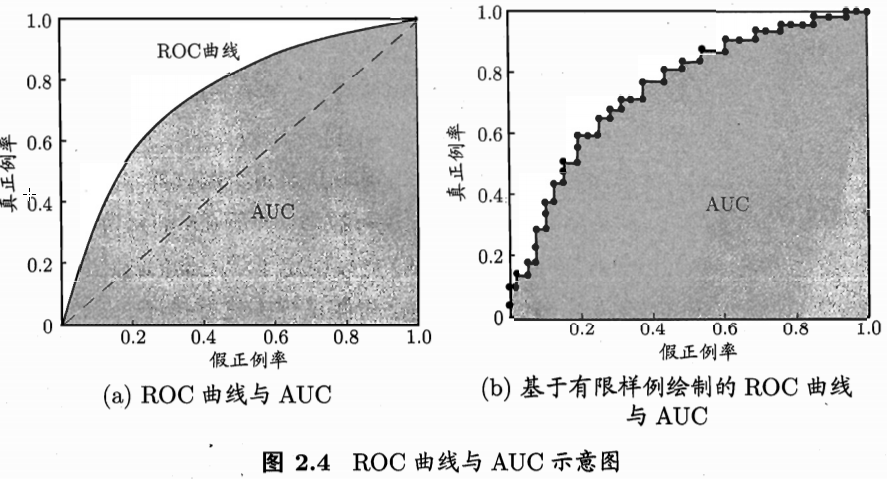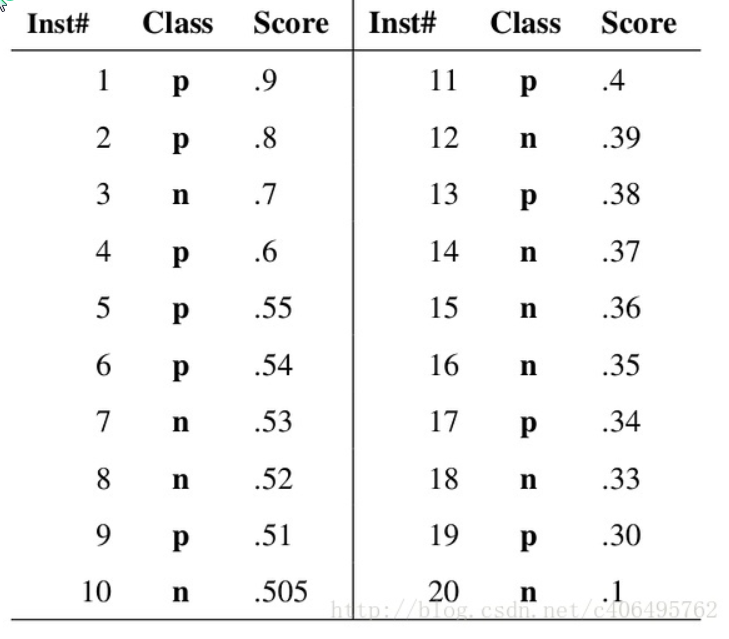# ROC与AUC曲线绘制

08/09 14:24(1) 在所排序的样本最左边，画一条线即  无 | 1 2 3 4 5 …，线左边的认为是正类，右边认为是负类，可以算出，TP(实际为正，预测为正)=0，FN(实际为正，预测为负)=10，TN(实际为负，预测为负)=10，FP(实际为负，预测为正)=0，故而TPR=0，FPR=0

(2) 在第一个样本后面，画一条线即 1 | 2 3 4 …，线左边的认为是正类，右边认为是负类，可以算出，TP(实际为正，预测为正)=1，FN(实际为正，预测为负)=9，TN(实际为负，预测为负)=10，FP(实际为负，预测为正)=0，故而TPR=0.1，FPR=0

(3) 在第二个样本后面，画一条线即 1 2| 3 4 …，线左边的认为是正类，右边认为是负类，可以算出，TP(实际为正，预测为正)=2，FN(实际为正，预测为负)=8，TN(实际为负，预测为负)=10，FP(实际为负，预测为正)=0，故而TPR=0.2，FPR=0

(4) 在第三个样本后面，画一条线即 1 2 3| 4 5…，线左边的认为是正类，右边认为是负类，可以算出，TP(实际为正，预测为正)=2，FN(实际为正，预测为负)=8，TN(实际为负，预测为负)=10，FP(实际为负，预测为正)=1，故而TPR=0.2，FPR=0.1

... ...

``````import numpy as np
import matplotlib.pyplot as plt
from matplotlib.font_manager import FontProperties

def plotROC(predStrengths,classLabels):
font = FontProperties(fname = r"c:\windows\fonts\simsun.ttc",size=14)
cur=(1.0,1.0)             #绘制光标位置
ySum=0.0                  #用于计算AUC
numPosClas=np.sum(np.array(classLabels)==1.0)  #正类样本的个数
yStep = 1/float(numPosClas)                    #Y轴步长
xStep = 1/float(len(classLabels)-numPosClas)   #X轴步长

sortedIndicies = predStrengths.argsort()
fig =plt.figure()
fig().clf()
ax=plt.figure()
for inddex in sortedIndicies.tolist():
if classLabels[index]==1.0
delX=0; delY = yStep   #碰到一个正类，Y的步长减 1
else:
delX = xStep; delY=0   #碰到一个负类，X的步长减1
ySum += cur         #每次cur都可能变化了       #高度累加
ax.plot([cur,cur-delX],[cur,cur-delY],c = 'b')   #绘制ROC
cur = (cur-delX,cur-delY)                         #更新绘制光标的位置
ax.plot([0,1],[0,1],'b--')
plt.title('ROC曲线'，FontProperties =font)
plt.xlabel('假正例率'，FontProperties = font)
plt.ylabel(('真正例率',FontProperties = font))
ax.axis([0,1,0,1])
print('AUC面积：',ySum*xStep)                         #计算AUC
plt.show()``````

0
0 收藏

### 作者的其它热门文章0 评论
0 收藏
0